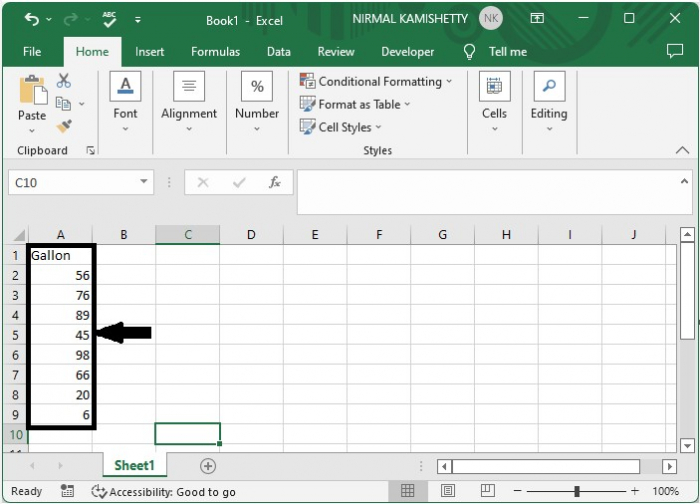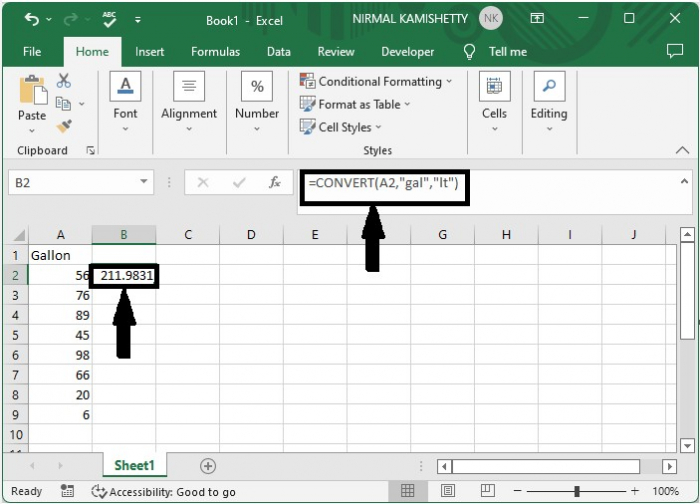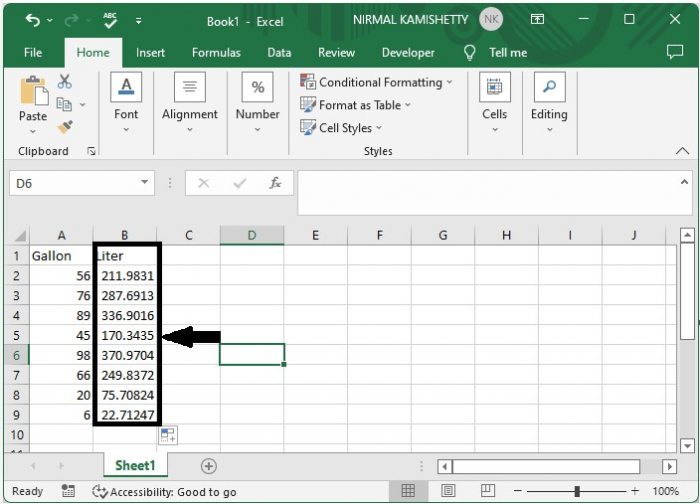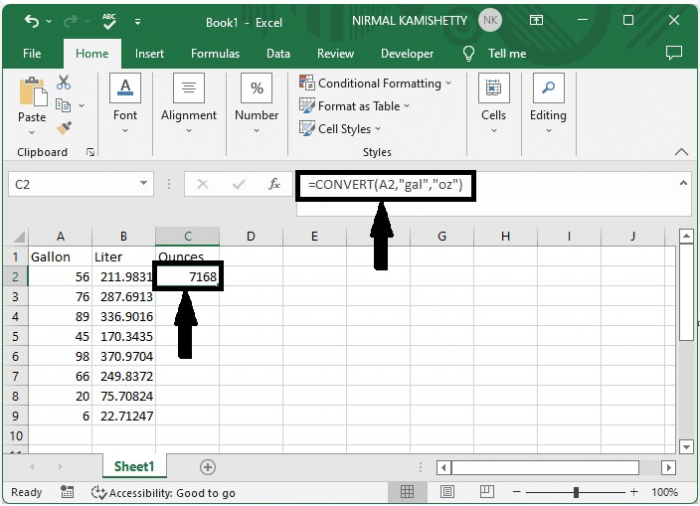# How to Convert Gallon to Liter/Ounce or Vice Versa in Excel?

Generally, we always try to measure a liquid in liters. It is the basic metric to measure liquids. Other metric units include ounces and gallon. When we measure in gallons, it is very important to change them into litres to make them more understandable to many users.

In this tutorial, we will learn how to convert a gallon to litres or ounces in Excel. The litre can be the most accurate unit to measure volume. We use simple shortcuts to complete our process.

## Convert Gallon to Liter/Ounce or Vice Versa Using Formulas

Here, we will first get any one of the results using the CONVERT formula, then use the auto-fill handle to get all the results. Let's go over a simple procedure for converting a gallon to litres or ounces in Excel using formulas.

### Step 1

Let us consider an Excel sheet that contains a list of values similar to the one shown below.Now to convert the gallon to liters, we can use the formula =CONVERT(A2,"gal","lt") and click Enter to get our first result.### Step 2

We can get all the other results by dragging them from the result cell using the auto-fill handle, and our final result will be similar to the below image.We can also use the formula =CONVERT(B2,"lt,"gal) to convert litres to gallon.

### Step 3

To convert gallons to ounces, click on an empty cell, in this case cell C2, and enter the formula =CONVERT(A2,"gal," "oz"), then press Enter.We use the formula =CONVERT(A2,"oz,"gal") to convert ounces to gallon.

Here, "lt" stands for liter, "oz" stands for ounce, and "gal" stands for gallon. We can use any combination to convert the values. The first value is the address of our value, the second value is our existing metric, and the third value is the metric we need.

## Conclusion

In this tutorial, we used a simple example to demonstrate how you can convert a gallon to a litre, ounce, or vice versa in Excel.

Updated on: 08-Feb-2023

592 Views